# The Best Way to Teach Multiplication Facts: 12 Steps to True UnderstandingTeaching multiplication is no easy task! Encouraging students to memorize the facts only allows a small percentage of them to be successful. Even then, those good memorizers may not truly understand what multiplication means. Rather than teaching multiplication for memorization, we want to teach it for understanding. Once understanding is built, it’s easier to become automatic with the facts!

If you are teaching multiplication for the first time, or if you’ve taught it before but have students who don’t seem to be grasping it, here are 12 simple steps to teaching multiplication for true understanding.

## STEP 1: SUBITIZE TO ENCOURAGE MULTIPLICATIVE REASONING

We can help our students develop multiplicative reasoning long before multiplication is even introduced. Subitizing with ten frames, tally marks, dot patterns, or base ten blocks can help students get a sense of what equal groups looks like. For example, the dice pattern below shows 8 equal groups of 3. We can figure out the total number of dots by adding 3+3+3+3+3+3+3+3 but this is also an opportunity to introduce language such as 8 groups of 3.

See more about subitizing for multiplication HERE or get these Multiplication Subitizing Cards HERE.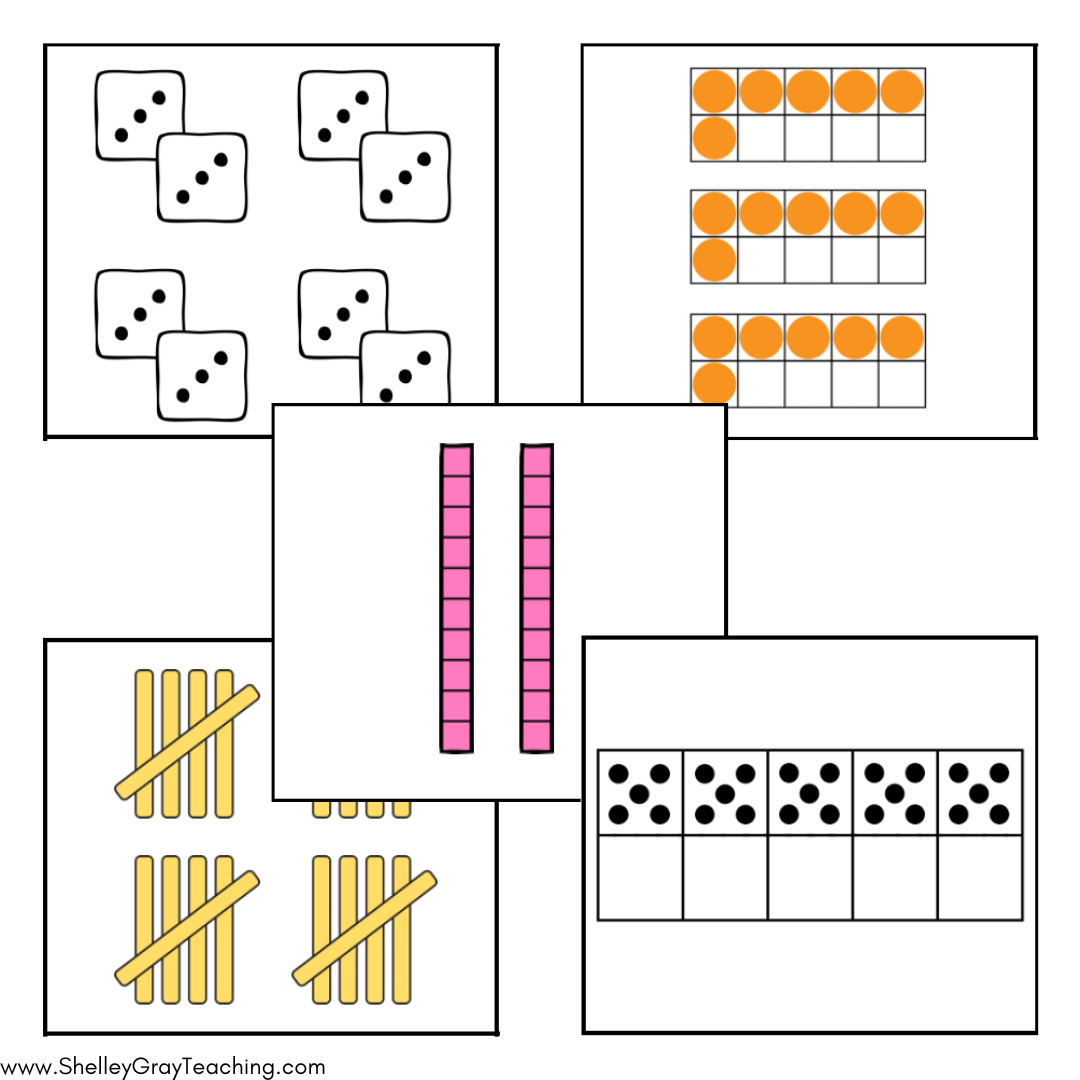## STEP 2: CONNECT MULTIPLICATION TO REPEATED ADDITION

Multiplication is a shortcut for addition, and we want our students to be able to see that connection. When we first teach multiplication, students must know that this is not a brand new foreign concept. It is simply an extension of what they already know. This will help multiplication seem less intimidating.

A problem-solving context is an effective way to help students see these connections. The problem below can be solved using addition, but we can also introduce multiplication as a potential method to solve it. Rather than introduce the times symbol immediately, we’ll start with equal groups.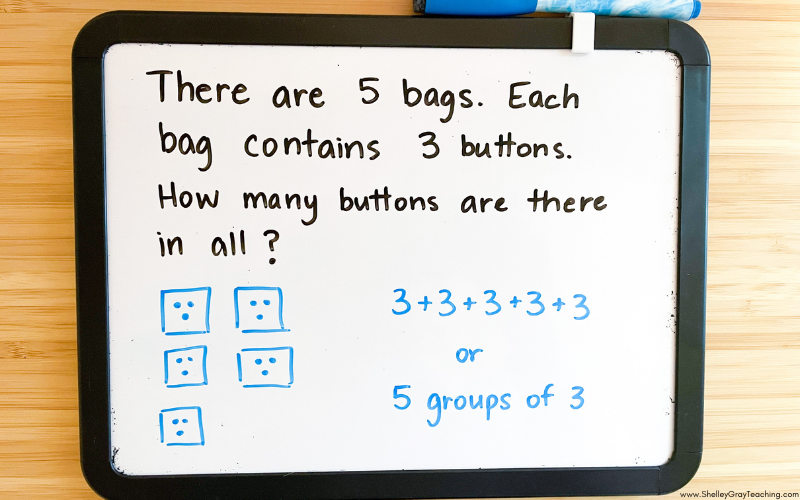Multiplication should be connected to repeated addition in a way that makes sense for students.

## STEP 3: EQUAL GROUPS

Students must understand multiplication as equal groups. If your students have already built some multiplicative reasoning (See Step 1), this will be easier to understand. Provide lots of opportunity for students to work with equal groups in a problem-solving context. This will ensure that these equal groups are a more concrete concept, rather than too abstract.

## STEP 4: SKIP COUNT

Skip counting is one of the beginning strategies for multiplication. We can skip count using equal groups and then transition to skip counting on a number line.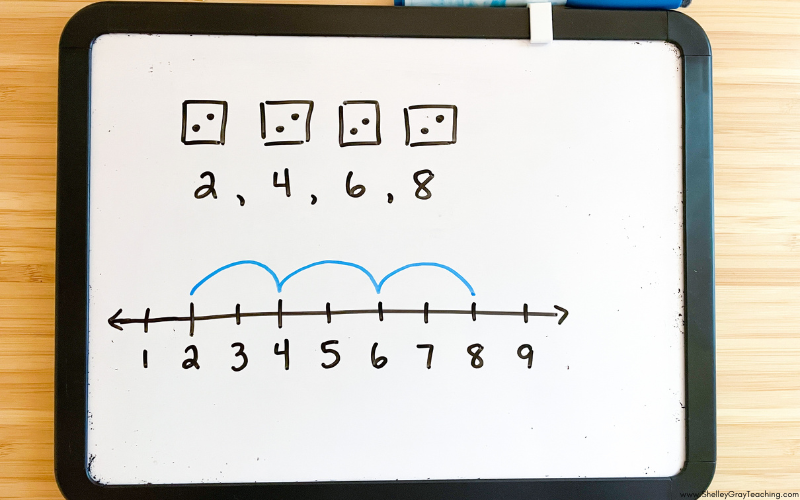## STEP 5: USE MANIPULATIVES

This step should be used through all of your multiplication teaching, but is so often missed when teachers go directly to multiplication worksheets involving pencils and paper only. Manipulatives provide rich experiences for your students to build their understanding of multiplication, and will ultimately allow more of your students to be successful. Any object can be used for students to create equal groups, such as coins, unifix cubes, base ten blocks, or any other counters you may have in your classroom.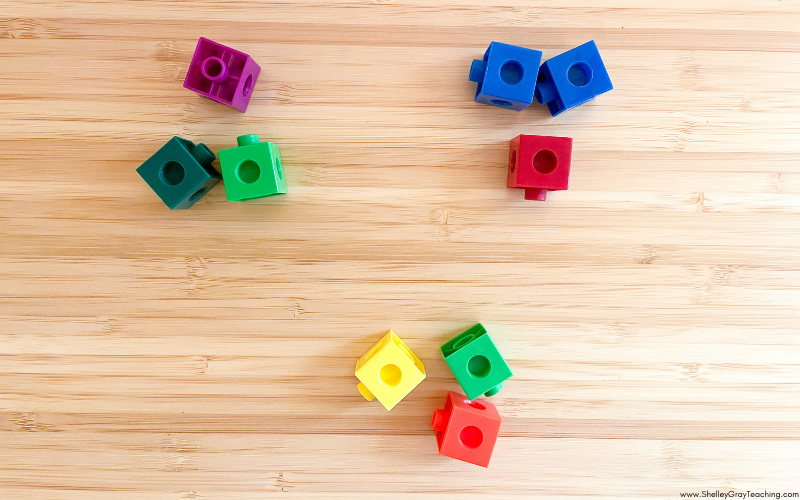One of my absolute favorite ways to have students represent multiplication is using a rekenrek, which you can read more about HERE.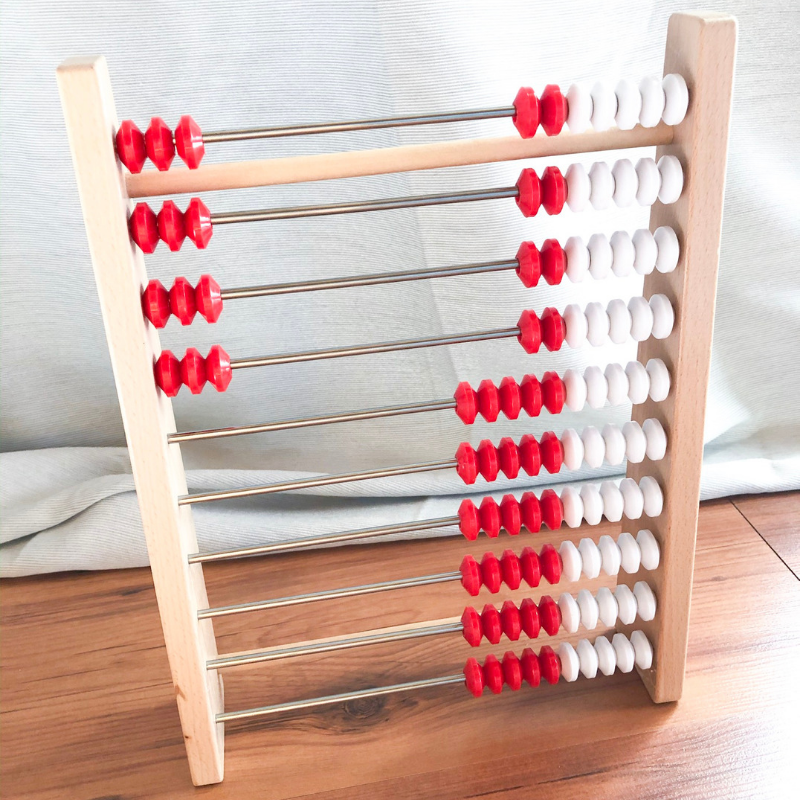## STEP 6: USE ARRAYS

Arrays are one of the most under-utilized tools for teaching multiplication.

We can use arrays to show students how different facts are connected. For example, the image below shows how 3×4 and 4×4 are connected. 4×4 is simply one more group of 4! Arrays can be built with physical objects (concrete) and then also drawn to represent that physical formation (representational).

RELATED: See more on the Concrete Representational Abstract Model for teaching math HERE.STEP 7: TEACH THE COMMUTATIVE PROPERTY

The number of multiplication facts students need to learn can be overwhelming, but it helps to understand the commutative property! When students understand that the product of 3×4 is the same as 4×3, it considerably decreases the number of facts we need to remember! Arrays are one of the best ways to show students the commutative property. Here we can see that when this 3 by 4 array is rotated, it becomes a 4 by 3 array!## STEP 8: MAKE MULTIPLICATION RELEVANT

Multiplication is not one of those concepts that students are never going to use – rather, multiplication will be used all the time in real life. By incorporating real-life scenarios, we can make multiplication relevant and more interesting to students. Real-world problem solving also helps students develop a more conceptual understanding because they can visualize what the multiplication problem really means. How can we do this? We can introduce problems, rather than simple expressions. We can talk about what the problem means. We can also use project-based learning that helps students make those real-life connections.

## STEP 9: HELP STUDENTS MAKE CONNECTIONS

Learning multiplication is so much easier when students realize the facts are connected and related to one another. 4×6 and 8×6 do not have to be understood as completely unrelated facts. Rather, 8×6 is simply the double of 4×6! This becomes apparent when we use pictures or visual models. Look at the arrays below. This makes it clear that if we double 4 groups of 6 (24) then we get 8 groups of 6 (48).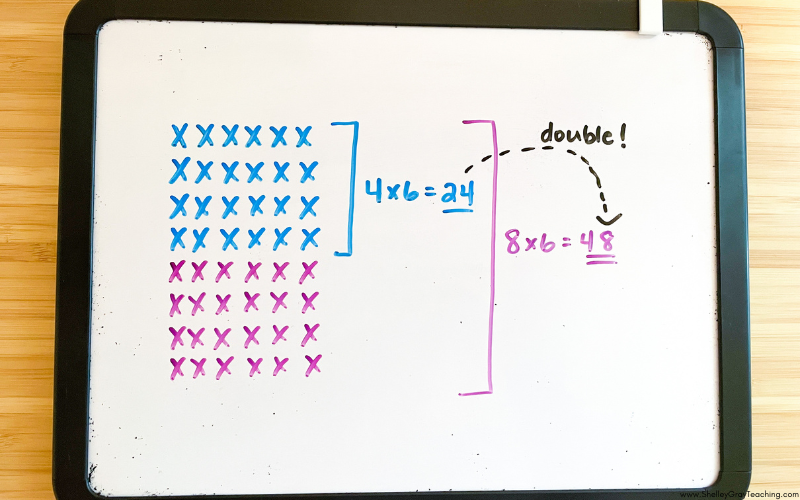These connections are EMPOWERING for students, because it means that they can literally solve anything!

## STEP 10: TEACH THE FACTS IN A STRATEGIC ORDER

When I first started teaching multiplication, I taught the facts in regular numerical order. Little did I know, this was completely WRONG! I was making multiplication more difficult than it had to be for my students. When you teach in a strategic order that makes practical sense, it helps students make connections between sets of facts. I recommend the following order of teaching:

x2

x10

x5

x1

x0

square facts (ex. 3×3, 4×4, 5×5, etc)

x4

x3

x9

x6

x7

x8

## STEP 11: TALK ABOUT MULTIPLICATION!

It’s important for students to see that there are many different ways to think about and solve problems. Often math is thought of as a black or white, right or wrong subject. But it can be fascinating to see that it’s actually a flexible subject that allows us to think in different ways! One of the best ways to give your students the opportunity to practice flexibility is by having them participate in number talks or math talks.

Through listening and sharing, students are exposed to different ways of thinking, strategizing, and reasoning. This is powerful.

Number talks can feel intimidating for teachers because can feel like you are not prepared enough. But that’s the beauty of it. The role of the teacher is to listen – listen to how students think, listen to how they explain, and listen to how they strategize.

We can learn so much by simply listening.

Here’s an example of a math talk you might have with your students. Think of all the opportunities for rich discussion with this slide. We can see how we double and then double again. We can see how 10×4 is half of 20×4. We can see how 20×4 is 4 times greater than 5×4. We can see that 20×4 has the same product as 10×8.

To get started with a math talk, simply draw a multiplication picture on the board and see where the conversation takes you! Or find out more about these Math Conversations slides, ready-made for the entire year HERE.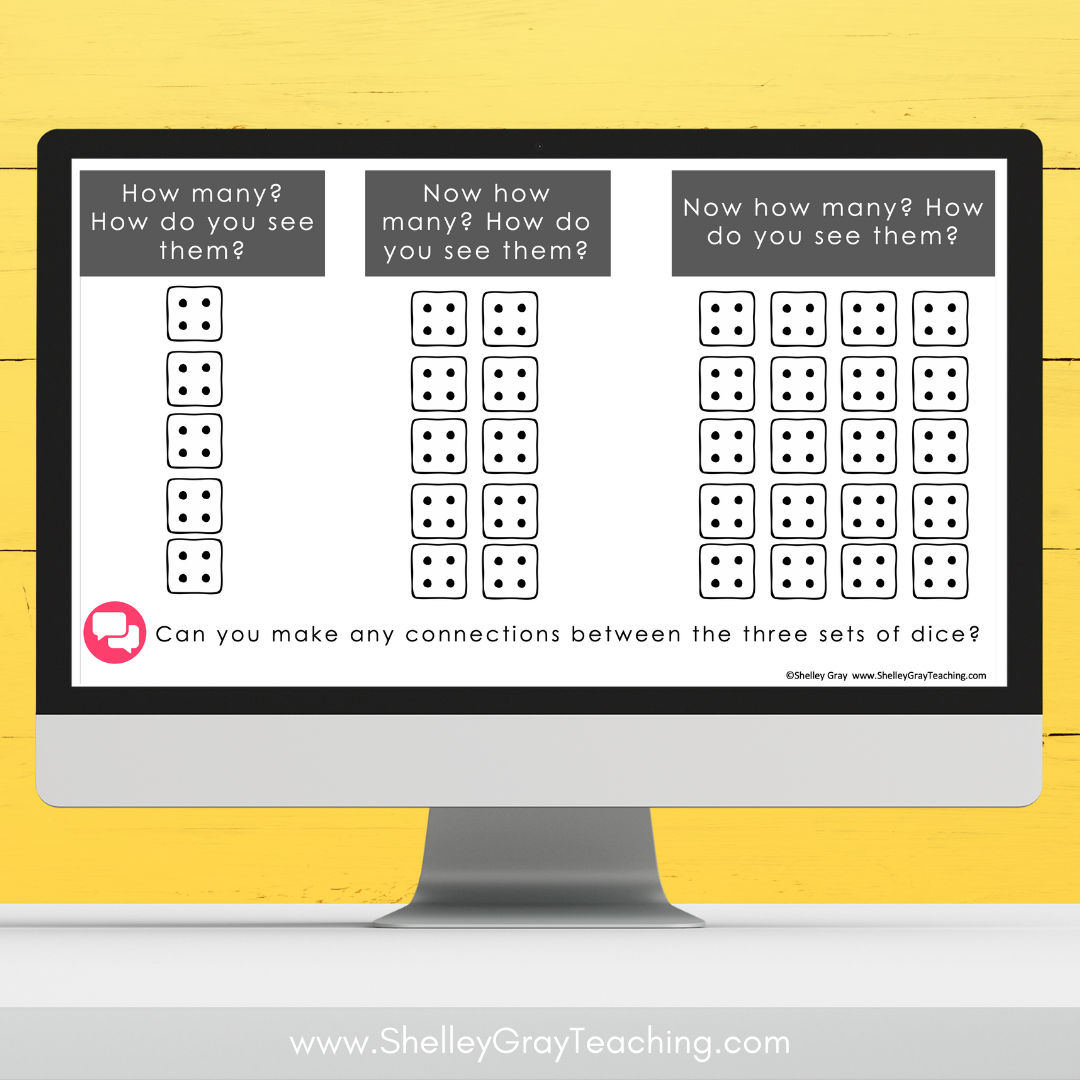## STEP 12: REINFORCE MULTIPLICATION ALL YEAR LONG

Multiplication is not just a “unit” that you teach and then move on to other things. Multiplication is an essential operation that is important to your students’ success in future grades. It is highly relevant to their real life and will be used in any job they have. Because of its importance to your students’ future success, multiplication (and the other basic operations) must be reinforced all year long.

This can be done by having a Math Facts center during your daily station time where students work on math fact understanding and fluency.

Here are some free math activities for your math facts center to get you started.

Regular reinforcement can also be done by playing games to practice math fact recall and by spiralling the curriculum and returning to those key concepts repeatedly.

I hope this article helps to empower you to teach multiplication for true understanding, rather than for memorization only. Although it may seem complex, you make a life-changing difference for your students by making small changes and beginning to implement the suggestions above.

If you’d like a completely done-for-you system for teaching multiplication, check out The Multiplication Station, used by tens of thousands of teachers. Or grab a free sample of it here!

This site uses Akismet to reduce spam. Learn how your comment data is processed.